# KSEEB Solutions for Class 10 Maths Chapter 9 Polynomials Ex 9.4

Students can Download Class 10 Maths Chapter 9 Polynomials Ex 9.4 Questions and Answers, Notes Pdf, KSEEB Solutions for Class 10 Maths helps you to revise the complete Karnataka State Board Syllabus and score more marks in your examinations.

## Karnataka State Syllabus Class 10 Maths Chapter 9 Polynomials Ex 9.4

Question 1.
Verify that the numbers given alongside of the cubic polynomials below are their zeroes. Also verify the relationship between the zeroes and the coefficients in each case:
(i) 2x3 + x2 – 5x + 2; $$\frac{1}{2}$$, 1, – 2
(ii) x3 – 4x2 + 5x – 2; 2, 1, 1
Let P(x) = 2x3 + x2 – 5x + 2 on comparing with general polynomial P(x) = ax3 + bx2 + cx + d we get
a = 2, b = 1, c = – 5 and d = 2
Given zeroes $$\frac{1}{2}$$, 1, – 2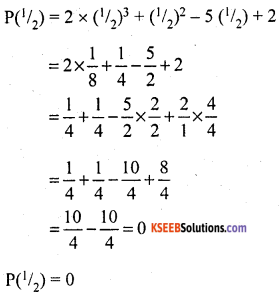P(1) = 2 (1)3 + (1)2 – 5(1) + 2
= 2 × 1 + 1 – 5 + 2
= 2 + 1 – 5 + 2 = 5 – 5 = 0
P(1) = 0
P(- 2) = 2(- 2)3 + (-2)2 – 5(- 2) + 2
= 2 × – 8 + 4 + 10 + 2
= – 16 + 16
P(- 2) = 0
Hence 1/2, 1 & -2 are the zeroes of the given cubic polynomial
Again consider α = 1/2, β = 1 & γ = – 2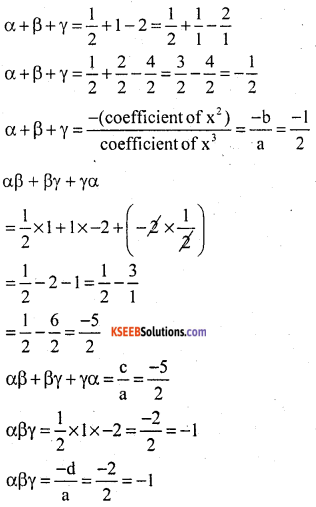ii) Let P(x) = x3 – 4x2 + 5x – 2 on comparing with general polynomial
P(x) = ax3 + bx2 + cx + d
we get a = 1, b = – 4, c = 5 & d = 2.
Given zeroes 2, 1, 1
P(2) = (2)3 – 4(2)2 + 5(2) – 2
= 8 – 4 × 4 + 10 – 2
= 8 – 16 + 10 – 2
P(2) = 18 – 18 = 0
P(2) = 0
P(1) = (1)3 – 4(1)2 + 5(1) – 2
= 1 – 4 + 5 – 2
P(1) = 6 – 6 = 0
P(1) = 0
Hence, 2, 1 and 1 are the zeroes of the given cubic polynomial.
Again, consider α = 2, β = 1 & γ = 1
α + β + γ = 2 + 1 + 1 = 4
and α + β + γ = $$\frac{-b}{a}=\frac{-(-4)}{1}$$ = 4
αβ + βγ + γα = 2(1) + (1) (1) + (1) × (2)
= 2 + 1 + 2 = 5
αβ + βγ + γα = $$\frac{c}{a}=\frac{5}{l}$$ = 5
αβγ = 2 × 1 × 1 = 2
αβγ = $$\frac{-d}{a}=\frac{-(-2)}{1}=\frac{2}{1}$$ = 2Question 2.
Find a cubic polynomial with the sum, sum of the product of its zeroes taken two at a time, and the product of its zeroes as 2, – 7, – 14 respectively.
Let the cubic polynomial be P(x) = ax3 + bx2 + cx + d
Then sum of zeroes = $$\frac{-b}{a}$$ = 2
Sum of the product of zeroes taken two at a time = $$\frac{c}{a}$$ = – 7
Product of zeroes = $$\frac{-d}{a}$$ = – 14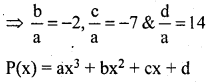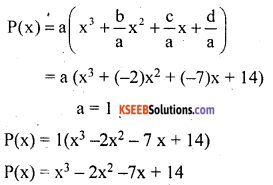Question 3.
If the zeroes of the polynomial x3 – 3x2 + x + 1 are a – b, a, a + b, find a and b.
The given Polynomial is x3 – 3x2 + x + 1
comparing with Ax3 + Bx2 + Cx + D,
A = 1, B = – 3, C = 1 & D = 1
Let α = a – b, β = a & γ = a + b
α + β + γ = $$\frac{B}{A}=\frac{-(-3)}{1}$$ = 3
a – b + a + a + b = 3
3a = 3
a = $$\frac{3}{3}$$ = 1
a = 1
αβγ = $$\frac{-D}{A}$$ = – 1
(a – b) a (a + b) = – 1 Put a = 1
(1 – b) 1 (1 + b) = – 1
1 – b2 = – 1
1 + 1 = b2
b2 = 2
b = ±$$\sqrt{2}$$
∴ a = 1 & b = ±$$\sqrt{2}$$Question 4.
If two zeroes of the polynomial x4 – 6x3 – 26x2 + 138x – 35 are 2 ±$$\sqrt{3}$$. find other zeroes.
Two zeroes of the given polynomial x4 – 6x3 – 26x2 + 138x – 35 are 2 ± $$\sqrt{3}$$.
∴ x = 2 ± $$\sqrt{3}$$.
Therefore [(x – (2 + $$\sqrt{3}$$)] [x – (2 – $$\sqrt{3}$$)]
= (x – 2 – $$\sqrt{3}$$) (x – 2 + $$\sqrt{3}$$)
= [(x – 2) – $$\sqrt{3}$$][(x – 2) + $$\sqrt{3}$$)]
= (x – 2)2 – ($$\sqrt{3}$$)2
= x2 – 4x + 4 – 3 = x2 – 4x + 1
∴ It is factor of Polynomial apply division algorithm.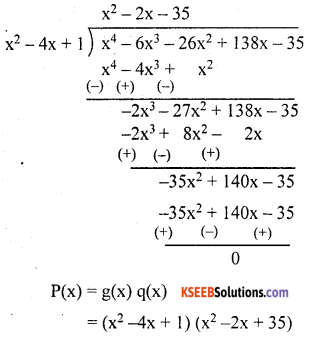Other two zeroes are given by the Quotient
x2 – 2x – 35 = 0
x2 – 7x + 5x – 35 = 0
x(x – 7) + 5(x – 7) = 0
(x – 7)(x + 5) = 0
x – 7 = 0 (or) x + 5 = 0
x = 7 (or) x = – 5
∴ x = 7, – 5

Question 5.
If the polynomial x4 – 6x3 + 16x2 – 25x + 10 is divided by another polynomial x2 – 2x + k, the remainder comes out to be x + a, find k and a.
Let us apply the division algorithm to the given Polynomial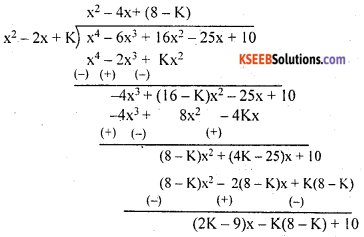But remainder is given to be x + a comparing coefficients of x in the remainder obtained and remainder given:
(2K – 9)x – K(8 – K)+ 10 = x + a
∴ 2K – 9 = 1
∴ 2K = 1 + 9
K = $$\frac{10}{2}$$
K = 5 and – K(8 – K) + 10 = a
Put K = 5
– 5(8 – 5) + 10 = a
– 5(3) + 10 = a
– 15 + 10 = a
a = – 5